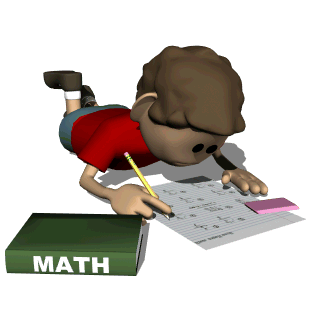•We are studying Geometry and angle measurements.

Eureka Student Workbook

Eureka Math Resources

Eureka Parent Resources

Percentage Calculator

Fraction Calculator

Visual Fractions

Fraction Action

Factorize

Factor Game

Fruit Splat

“I Can” Common Core!

I Can Use the Four Operations (+, -, x, ) to Help Me Understand Math

 I can understand that multiplication fact problems can be seen as comparisons of groups (e.g., 24 = 4 x 6 can be thought of as 4 groups of 6 or 6 groups of 4). 4.OA.1

 I can multiply or divide to solve word problems by using drawings or writing equations and solving for a missing number. 4.OA.2

 I can use what I know about addition, subtraction, multiplication and division to solve multi-step word problems involving whole numbers. 4.OA.3

 I can represent word problems by using equations with a letter standing for the unknown number. 4.OA.3

 I can determine how reasonable my answers to word problems are by using estimation, mental math and rounding. 4.OA.3

 I can find all factor pairs for a number from 1 to 100. 4.OA.4
 I can determine whether a given whole number up to 100 is a prime or composite number. 4.OA.4

 I can create a number or shape pattern that follows a given rule. 4.OA.5
 I can notice different features of a pattern once it is created by a rule. 4.OA.5

I Can Use Number Sense and Place Value to Help Me Understand Math

 I can recognize that in multi-digit whole number, a digit in one place represents ten times what it represents in the place to its right. 4.NBT.1

 I can read and write larger whole numbers using numerals, words and in expanded form. 4.NBT.2

 I can compare two large numbers using symbols to show the comparison. 4.NBT.2

 I can round large whole numbers to any place. 4.NBT.3

 I can add and subtract large numbers. 4.NBT.4

 I can multiply a whole number up to four digits by a one-digit whole number. 4.NBT.5

 I can multiply two two-digit numbers. 4.NBT.5
 I can find whole-number quotients and remainders with up to four-digit dividends and one-digit divisors. 4.NBT.6

I Can Use Fractions to Help Me Understand Math

 I can explain (and show models for) why multiplying a numerator and a denominator by the same number does not change the value of a fraction. 4.NF.1

 I can compare two fractions with different numerators and different denominators by creating common denominators or numerators or by comparing them to a benchmark fraction like one-half. 4.NF.2

 I can recognize that comparisons of fractions are valid only when the two fractions refer to the same whole. 4.NF.2

 I can compare fractions using symbols and justify the comparison by using models. 4.NF.2

 I can understand that improper fractions have a greater numerator than denominator. 4.NF.3

 I can understand addition and subtraction of fractions as joining and separating parts referring to the same whole. 4.NF.3

 I can decompose a fraction into a sum of fractions with the same denominator. 4.NF.3

 I can add and subtract mixed numbers with like denominators. 4.NF.3

 I can solve word problems involving addition and subtraction of fractions with like denominators. 4.NF.3

 I can multiply a fraction by a whole number. 4.NF.4
 I can solve word problems involving multiplication of a fraction by a whole number. 4.NF.4

 I can show a fraction with a denominator of 10 as an equivalent fraction with a denominator of 100 in order to add the two fractions. 4.NF.5

 I can use decimals to show fractions with denominators of 10 and 100. 4.NF.6  I can compare two decimals to hundredths by reasoning about their size. 4.NF.7

I Can Use Measurement and Data to Help Me Understand Math

 I can show that I know the relative size of measurement units within a single system. 4.MD.1

 I can show the measurements of a larger unit in terms of smaller units and record these in a table. 4.MD.1

 I can use the four operations (+, -, x, ) to solve word problems involving measurement; including simple fractions and decimals. 4.MD.2

 I can use what I know about area and perimeter to solve real world problems involving rectangles. 4.MD.3

 I can make a line plot to show measurements involving fractions. 4.MD.4  I can solve problems involving addition and subtraction of fractions by using information presented in line plots. 4.MD.4

 I can recognize angles as geometric shapes where two rays share a common endpoint. 4.MD.5

 I can understand that angles are measured with reference to a circle, with its center at the common endpoint of the rays. 4.MD.5

 I can use a protractor to measure angles in whole-number degrees. 4.MD.6  I can solve addition and subtraction problems involving angles. 4.MD.7

I Can Use Geometry to Help Me Understand Math

 I can identify and draw points, lines, line segments, rays, angles and perpendicular & parallel lines. 4.G.1

 I can classify two-dimensional shapes based on what I know about their geometrical attributes. 4.G.2

 I can recognize and identify right triangles. 4.G.2

 I can recognize and draw lines of symmetry. 4.G.3